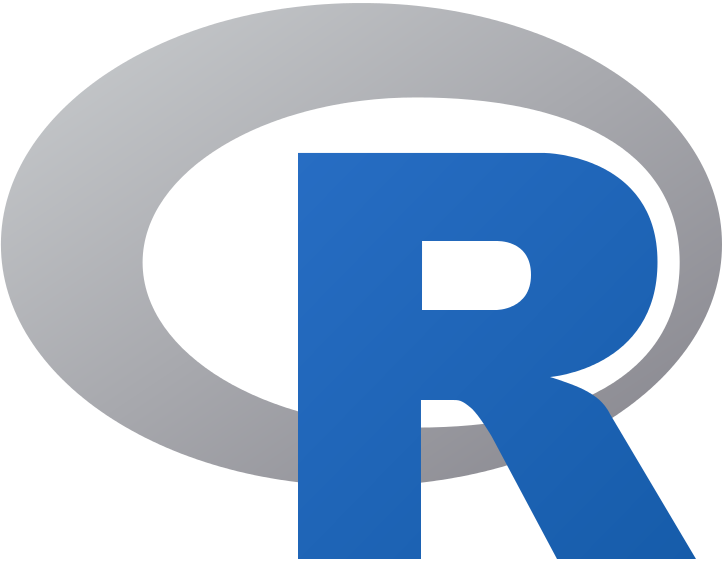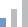Vissza

# R Programming from the Ground Up

## R Programming from the Ground Up

Kód: WA2711Időtartam:2 nap
Nehézségi szint:
•Kezdő
30 950 Ft
(Bruttó ár: 39 307 Ft)
Kérdésem van!
Képzési forma
e-learning
Képzés nyelve
angol

### Tájékoztató

Over the past few years, R has been steadily gaining popularity with business analysts, statisticians and data scientists as a tool of choice for conducting statistical analysis of data as well as supervised and unsupervised machine learning.

This intensive training course helps students learn the practical aspects of the R programming language. The course is supplemented by many hands-on labs which allow attendees to immediately apply their theoretical knowledge in practice.

### Termékleírás

This product is delivered as a voucher. After ordering, the voucher(s) will be available in your Dashboard (myLeapest). You will also receive required software set up for install 48 hours from time of purchase.

Content

The following contents are included with this product.

• R Programming from the Ground Up - Lecture Book - eBook-English-(en-US)
• R Programming from the Ground Up - Lab Guide

TOPICS

• High octane introduction to R programming
• Learning about R data structures
• Working with R functions
• Statistical data analysis with R

### Kiknek ajánljuk

Business Analysts, Technical Managers, and Programmers

### Szükséges előképzettség

Participants should have the general knowledge of statistics and programming

### Tematika

Outline of R Programming from the Ground Up Training

Chapter 1. What is R

• What is R?
• Positioning of R in the Data Science Space
• The Legal Aspects
• Microsoft R Open
• R Integrated Development Environments
• Running R
• Running RStudio
• Getting Help
• General Notes on R Commands and Statements
• Assignment Operators
• R Core Data Structures
• Assignment Example
• R Objects and Workspace
• Printing Objects
• Arithmetic Operators
• Logical Operators
• System Date and Time
• Operations
• User-defined Functions
• Control Statements
• Conditional Execution
• Repetitive Execution
• Repetitive execution
• Built-in Functions
• Summary

Chapter 2. Introduction to Functional Programming with R

• What is Functional Programming (FP)?
• Terminology: Higher-Order Functions
• A Short List of Languages that Support FP
• Functional Programming in R
• Vector and Matrix Arithmetic
• Vector Arithmetic Example
• More Examples of FP in R
• Summary

Chapter 3. Managing Your Environment

• Getting and Setting the Working Directory
• Getting the List of Files in a Directory
• The R Home Directory
• Executing External R commands
• Loading External Scripts in RStudio
• Listing Objects in Workspace
• Removing Objects in Workspace
• Saving Your Workspace in R
• Saving Your Workspace in RStudio
• Saving Your Workspace in R GUI
• Loading Your Workspace
• Diverting Output to a File
• Batch (Unattended) Processing
• Controlling Global Options
• Summary

Chapter 4. R Type System and Structures

• The R Data Types
• System Date and Time
• Formatting Date and Time
• Using the mode() Function
• R Data Structures
• What is the Type of My Data Structure?
• Creating Vectors
• Logical Vectors
• Character Vectors
• Factorization
• Multi-Mode Vectors
• The Length of the Vector
• Getting Vector Elements
• Lists
• A List with Element Names
• Extracting List Elements
• Adding to a List
• Matrix Data Structure
• Creating Matrices
• Creating Matrices with cbind() and rbind()
• Working with Data Frames
• Matrices vs Data Frames
• A Data Frame Sample
• Creating a Data Frame
• Accessing Data Cells
• Getting Info About a Data Frame
• Selecting Columns in Data Frames
• Selecting Rows in Data Frames
• Getting a Subset of a Data Frame
• Sorting (ordering) Data in Data Frames by Attribute(s)
• Editing Data Frames
• The str() Function
• Type Conversion (Coercion)
• The summary() Function
• Checking an Object's Type
• Summary

Chapter 5. Extending R

• The Base R Packages
• Loading Packages
• What is the Difference between Package and Library?
• Extending R
• The CRAN Web Site
• Extending R in R GUI
• Extending R in RStudio
• Installing and Removing Packages from Command-Line
• Summary

Chapter 6. Read-Write and Import-Export Operations in R

• Reading Data from a File into a Vector
• Example of Reading Data from a File into A Vector
• Writing Data to a File
• Example of Writing Data to a File
• Reading Data into A Data Frame
• Writing CSV Files
• Importing Data into R
• Exporting Data from R
• Summary

Chapter 7. Statistical Computing Features in R

• Statistical Computing Features
• Descriptive Statistics
• Basic Statistical Functions
• Examples of Using Basic Statistical Functions
• Non-uniformity of a Probability Distribution
• Writing Your Own skew and kurtosis Functions
• Generating Normally Distributed Random Numbers
• Generating Uniformly Distributed Random Numbers
• Using the summary() Function
• Math Functions Used in Data Analysis
• Examples of Using Math Functions
• Correlations
• Correlation Example
• Testing Correlation Coefficient for Significance
• The cor.test() Function
• The cor.test() Example
• Regression Analysis
• Types of Regression
• Simple Linear Regression Model
• Least-Squares Method (LSM)
• LSM Assumptions
• Fitting Linear Regression Models in R
• Example of Using lm()
• Confidence Intervals for Model Parameters
• Example of Using lm() with a Data Frame
• Regression Models in Excel
• Multiple Regression Analysis
• Summary

Chapter 8. Data Manipulation and Transformation in R

• Applying Functions to Matrices and Data Frames
• The apply() Function
• Using apply()
• Using apply() with a User-Defined Function
• apply() Variants
• Using tapply()
• Adding a Column to a Data Frame
• Dropping A Column in a Data Frame
• The attach() and detach() Functions
• Sampling
• Using sample() for Generating Labels
• Set Operations
• Example of Using Set Operations
• The dplyr Package
• Object Masking (Shadowing) Considerations
• Getting More Information on dplyr in RStudio
• The search() or searchpaths() Functions
• Handling Large Data Sets in R with the data.table Package
• The fread() and fwrite() functions from the data.table Package
• Using the Data Table Structure
• Summary

Chapter 9. Data Visualization in R

• Data Visualization
• Data Visualization in R
• The ggplot2 Data Visualization Package
• Creating Bar Plots in R
• Creating Horizontal Bar Plots
• Using barplot() with Matrices
• Using barplot() with Matrices Example
• Customizing Plots
• Histograms in R
• Building Histograms with hist()
• Example of using hist()
• Pie Charts in R
• Examples of using pie()
• Generic X-Y Plotting
• Examples of the plot() function
• Dot Plots in R
• Saving Your Work
• Supported Export Options
• Plots in RStudio
• Saving a Plot as an Image
• Summary

Chapter 10. Using R Efficiently

• Object Memory Allocation Considerations
• Garbage Collection
• Finding Out About Loaded Packages
• Using the conflicts() Function
• Getting Information About the Object Source Package with the pryr Package
• Using the where() Function from the pryr Package
• Timing Your Code
• Timing Your Code with system.time()
• Timing Your Code with System.time()
• Sleeping a Program
• Handling Large Data Sets in R with the data.table Package
• Passing System-Level Parameters to R
• Summary

Lab Exercises

Lab 1. Getting Started with R
Lab 2. Learning the R Type System and Structures
Lab 3. Read and Write Operations in R
Lab 4. Data Import and Export in R
Lab 5. k-Nearest Neighbors Algorithm
Lab 6. Creating Your Own Statistical Functions
Lab 7. Simple Linear Regression
Lab 8. Monte-Carlo Simulation (Method)
Lab 9. Data Processing with R
Lab 10. Using R Graphics Package
Lab 11. Using R Efficiently

Megosztás

## Hírlevél feliratkozás

Az Adatvédelmi szabályzatot megértettem és elfogadom, feliratkozom a Számalk hírlevelére.